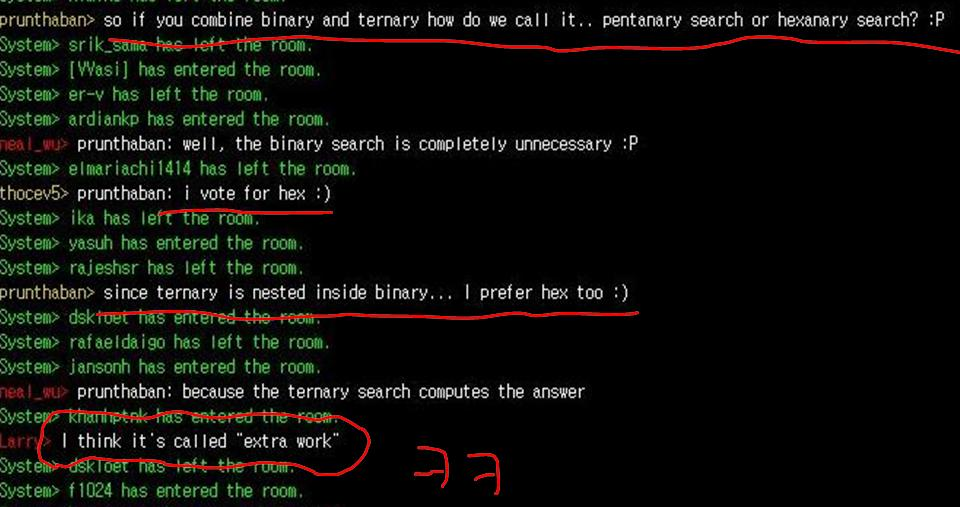## 탑코더 스름 426 디비젼 1

토->일 넘어가는 새벽 2시에 열린 매치.. 이번 매치는 정말 극악의 매치였다..~
특히 한국사람들은.. 새벽 두시에 졸음을 참으며 열심히 문제를 풀어야했고..
챌린지패이스에서 문제가 생기는바람에 시스템 테스트도 한참 늦게 해줘서..
채점 기다리느라 다들 고생이 많았다..;; 나도 덕분에 날밤 까고 말았다.. =_=;;;
게다가 250점 문제는 쉬운 문제임에도 불구하고 문제 디스크립션이 너무 엉망이라서..
대부분의 사람들이 낮은 코딩점수를 받았다.. (해석을 늦게해서.. -_-;;) 아.. 문제 출제자.. 좀 너무한거 아녀..~
이번 매치에서는 우리방에 챌이 하나도 없었다.. 시도한 사람조차 없었다..;;
남들 코드가 좀 의심가기는 하지만.. 마땅히 fail시킬 수 있는 케이스를 찾는것도 넘 힘들고..
좀 재미없었던 매치..시스템 테스트도 안되고있고.. 해서 사람들이 매치 끝나고 다들 어드민 방에 모여서 잡담을 하며 놀기도 했다..Level1 - ShufflingMachine
N개의 카드가 있고.. 이 카드를 섞는데.. 섞는 규칙은 shuffle 이라는 1부터 N까지 수의 permutation에 따른다.. 그러나 몇번 섞는지는 알 수 없고 최소 1번에서 최대 maxShuffle번을 섞는다.. 그리고나서 카드를 분배하게되는데.. 나는 cardReceived 번째의 카드들을 받게 된다.. 내가 원하는 카드가 K개이고 맨 처음 모든 카드의 순서를 내 맘대로 바꿀 수 있다.. 내가 받을 수 있는 원하는 카드의 기대값 구하기..

우선 1부터 maxShuffle번까지의 permutation을 다 구한다.. 그중에서 cardReceived column에 있는 수들만 쭉 읽어서 가장 많이 나온 수를 K개 고르면 된다.. 그 수들을 처음에 내가 원하는 곳에 위치시킬 수 있으므로..

코딩은 의외로 깔끔했는데.. 문제 해석이 오래걸려서 125점에 그쳤다..

1 #include <iostream>
2 #include <cstdio>
3 #include <algorithm>
4 #include <vector>
5 #include <string>
6 using namespace std;
7
8 int comp(const void* x, const void* y)
9 {
10     int* a = (int*)x;
11     int* b = (int*)y;
12     return *b - *a;
13 }
14
15 class ShufflingMachine {
16 public:
17
18 double stackDeck(vector <int> shuffle, int maxShuffles, vector <int> cardsReceived, int K)
19 {
20     int m, n;
21     int i, j, sum;
22     int perm;
23     int cnt;
24     m = shuffle.size();
26     for (i = 0; i < m; i++) {
27         perm[i] = i;
28     }
29     for (i = 1; i < maxShuffles; i++) {
30         for (j = 0; j < m; j++) {
31             perm[i][shuffle[j]] = perm[i-1][j];
32         }
33     }
34     memset(cnt, 0, sizeof(cnt));
35     for (i = 0; i < maxShuffles; i++) {
36         for (j = 0; j < n; j++) {
38         }
39     }
40     qsort(cnt, 200, sizeof(int), comp);
41     sum = 0;
42     for (i = 0; i < K; i++) {
43         sum += cnt[i];
44     }
45     return (double)sum / (double)maxShuffles;
46 }
47
48 };

Level2 - CatchTheMice
쥐들의 x, y 좌표가 주어지고 x방향 y방향 속도가 각각 주어진다.. t초 후의 번 쥐의 위치는 (xp[i]+xv[i]*t, yp[i]+yv[i]*t) 가 된다. 이때, 모든 순간에 대해서 모든 쥐를 다 포함할 수 없는 가장 큰 직사각형 구하기.. 경계선에 걸치는것은 포함되지 않는것으로 간주..

이 문제는 ternary search를 이용해서 풀 수 있다..
예를들어 쥐들이 처음에는 좀 멀리 떨어져있다가 시간이 흘러서 어느 특정 시점에 서로 가장 가까워지고
그 시점이 지나면 다시 멀어진다는 가정이 맞아야 한다..
직관적으로 생각해보면 맞는것 같기도하고.. ㅇ.ㅇ;;

따라서  f(x) = 시간 x에 대해 모든 쥐를 잡을 수 있는 가장 작은 사각형의 side
라고 정의하면 f(x)는 unimodal 형태가 되므로 ternary search 적용이 가능하다..

ternary search에 대한 더 자세한 내용은 알고스팟 강좌를 참조하자..

1 #include <iostream>
2 #include <cstdio>
3 #include <algorithm>
4 #include <vector>
5 #include <string>
6 using namespace std;
7 #define INF 999999999.0
8 #define min(x, y) ((x) > (y) ? (y) : (x))
9 #define max(x, y) ((x) > (y) ? (x) : (y))
10
11 typedef struct _p {
12     double x, y;
13 } POINT;
14
15 POINT pt, dt;
16 int n;
17
18 double f(double p)
19 {
20     double minx, miny, maxx, maxy;
21     double x, y;
22     int i;
23     minx = miny = INF;
24     maxx = maxy = -INF;
25     for (i = 0; i < n; i++) {
26         x = pt[i].x + dt[i].x * p;
27         y = pt[i].y + dt[i].y * p;
28         minx = min(minx, x);
29         miny = min(miny, y);
30         maxx = max(maxx, x);
31         maxy = max(maxy, y);
32     }
33     return max(maxx-minx, maxy-miny);
34 }
35 double t_search(double left, double right)
36 {
37     int it;
38     double a, b;
39     it = 200;
40     while (it--) {
41         a = (left * 2.0 + right) / 3.0;
42         b = (left + right * 2.0) / 3.0;
43
44         /* f(): 감소 -> 증가 */
45         if (f(a) > f(b))
46             left = a;
47         else
48             right = b;
49     }
50     return f((left + right) * 0.5);
51 }
52
53 class CatchTheMice {
54 public:
55
56 double largestCage(vector<int> xp, vector<int> yp, vector<int> xv, vector<int> yv)
57 {
58     int i;
59     n = xp.size();
60     for (i = 0; i < n; i++) {
61         pt[i].x = xp[i];
62         pt[i].y = yp[i];
63         dt[i].x = xv[i];
64         dt[i].y = yv[i];
65     }
66     return t_search(0, 1000000);
67 }
68
69 };